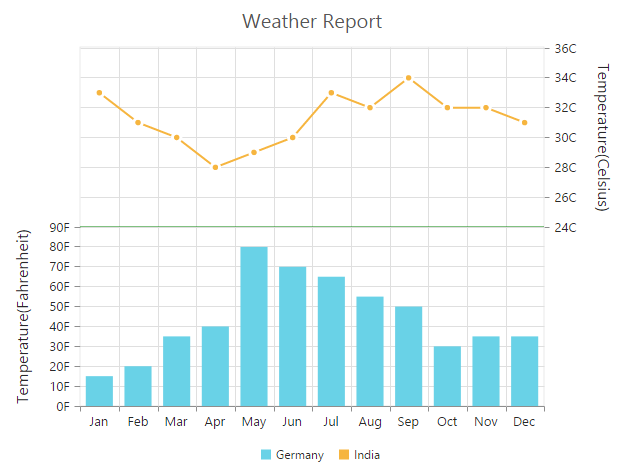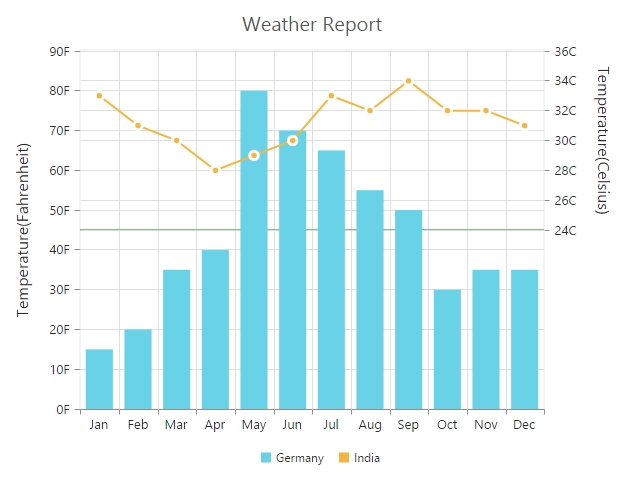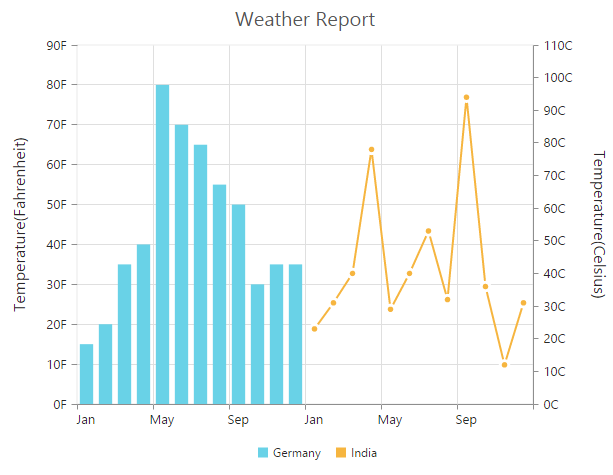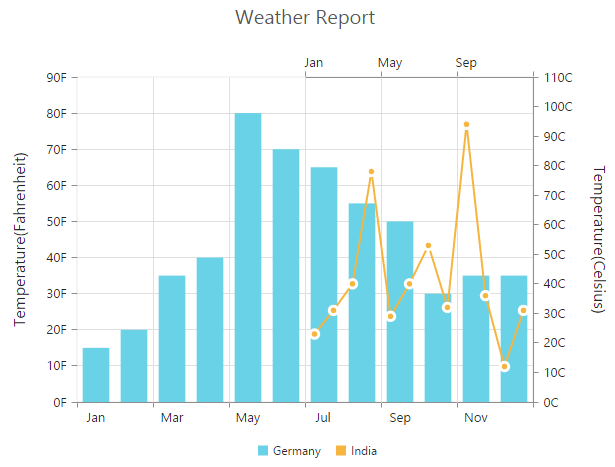# Multiple panes

6 Jul 20165 minutes to read

Chart area can be divided into multiple panes using the RowDefinitions and ColumnDefinitions properties.

### Row Definitions

To split the chart area vertically into a number of rows, use RowDefinitions of the chart.

• You can allocate space for each row by using the Unit option that determines whether the chart area should be split by percentage or pixels for the given RowHeight value of the RowDefinitions.

• To associate a vertical axis to a row, specify the RowDefinitions Index value to the RowIndex property of the chart axis.

• To customize each row’s horizontal line, use LineColor and LineWidth property.

• HTML
• ``````<ej:Chart ID="Chart1" runat="server">
<%--Splitting chart area into multiple rows--%>
<RowDefinitions>
<%--Split first row of the chart area--%>
<ej:RowDefinitions Unit="percentage" LineColor="grey" LineWidth="0" RowHeight="50" />
<%--Split second row of the chart area--%>
<ej:RowDefinitions Unit="percentage" LineColor="green" LineWidth="0" RowHeight="50" />
</RowDefinitions>

<Axes>
<%--Create secondary axis and bind it to second row of chart area--%>
<ej:Axis Name="yAxis1" RowIndex="1">
</ej:Axis>
</Axes>

<Series>
<%--Binding vertical axis name--%>
<ej:Series YAxisName="yAxis1">
</ej:Series>
</Series>
</ej:Chart>``````Row Span

For spanning the vertical axis along multiple panes vertically, you can use RowSpan property of axis.

• HTML
• ``````<ej:Chart ID="Chart1" runat="server">
<%--Splitting chart area into multiple rows--%>
<RowDefinitions>
</RowDefinitions>

<Axes>
<%--Create secondary axis and bind it to second row of chart area--%>
</Axes>

<PrimaryYAxis RowSpan="2"></PrimaryYAxis>

<Series>
</Series>
</ej:Chart>``````## Column Definitions

To split the chart area horizontally into a number of columns, use ColumnDefinitions of the chart.

• You can allocate space for each column by using the Unit option that determines whether the chart area should be split by percentage or pixels for the given ColumnWidth value of the ColumnDefinitions.

• To associate a horizontal axis to a column, specify the ColumnDefinitions Index value to the ColumnIndex property of the chart axis.

• HTML
• ``````<ej:Chart ID="Chart1" runat="server">
<%--Splitting chart area into multiple columns--%>
<ColumnDefinitions>
<%--Split first column of the chart area--%>
<ej:ColumnDefinitions Unit="percentage" ColumnWidth="50"/>
<%--Split second column of the chart area--%>
<ej:ColumnDefinitions Unit="percentage" ColumnWidth="50"/>
</ColumnDefinitions>

<Axes>
<%--Create secondary axis and bind it to second row of chart area--%>
<ej:Axis Name="xAxis1" ColumnIndex="1">
</ej:Axis>
</Axes>

<Series>
<%--Binding horizontal axis name--%>
<ej:Series XAxisName="xAxis1">
</ej:Series>
</Series>
</ej:Chart>``````Column Span

For spanning the horizontal axis along multiple panes horizontally, you can use ColumnSpan property of axis.

• HTML
• ``````<ej:Chart ID="Chart1" runat="server">
<%--Splitting chart area into multiple columns--%>
<ColumnDefinitions>
<%-- split no.of column--%>
</ColumnDefinitions>

<Axes>